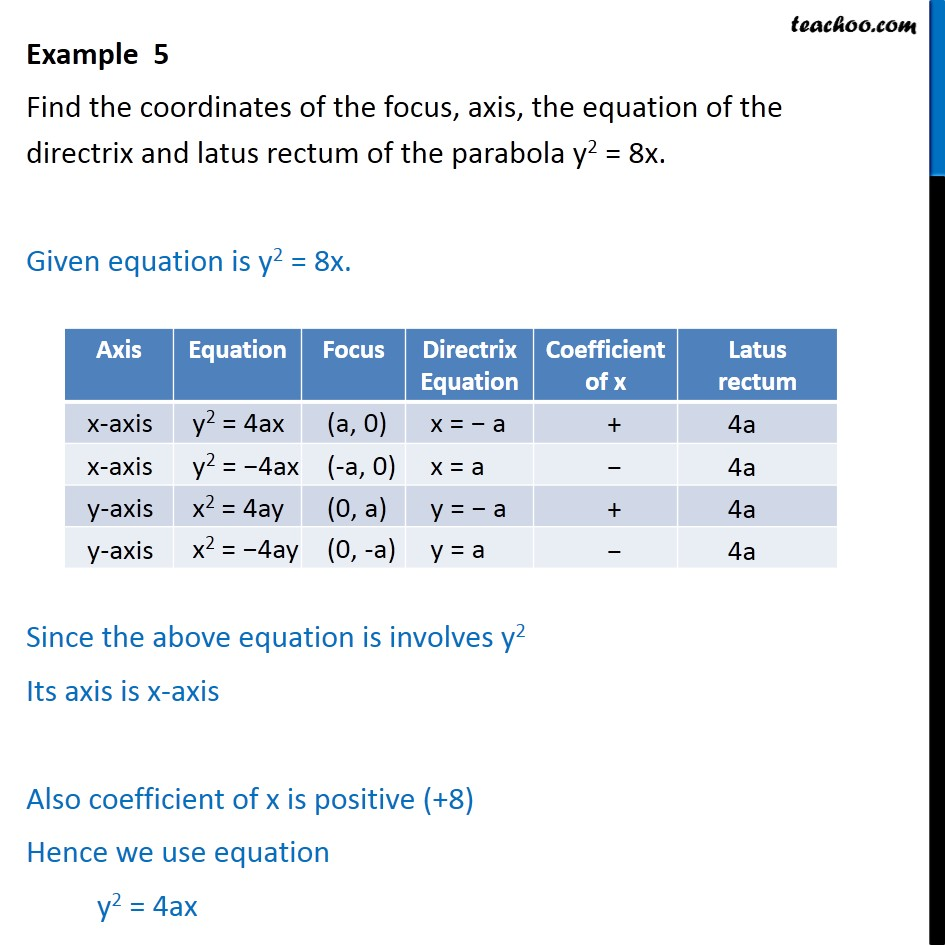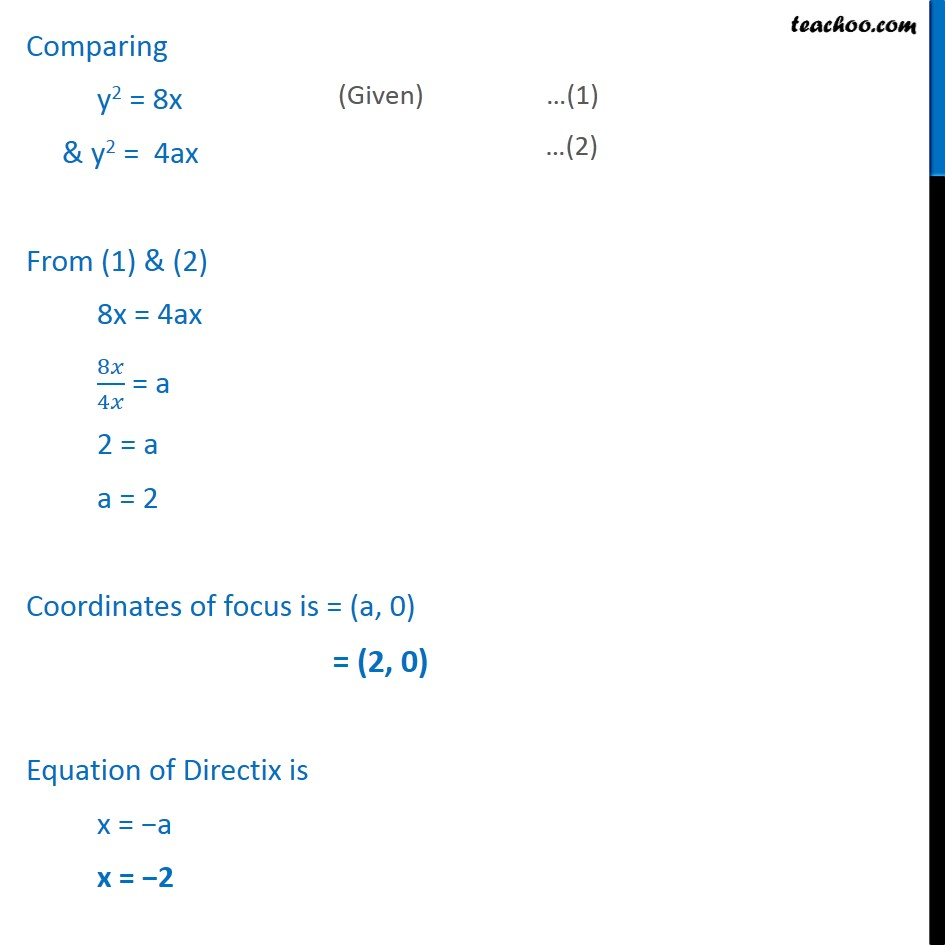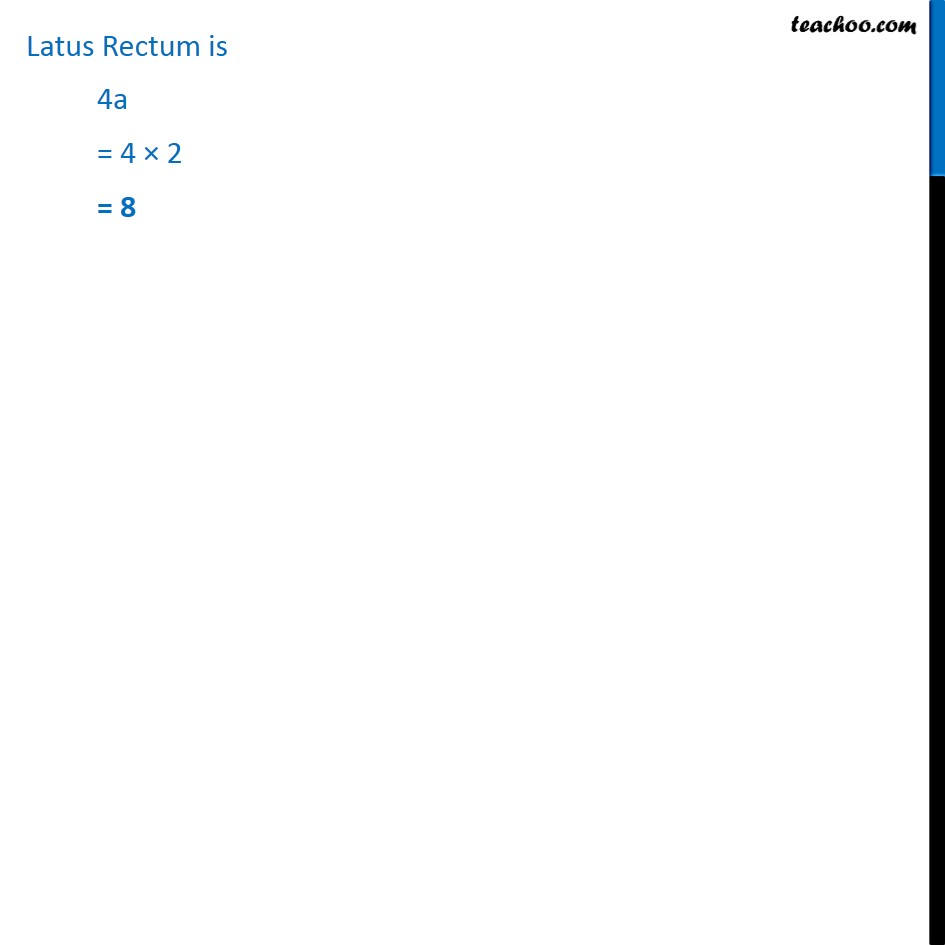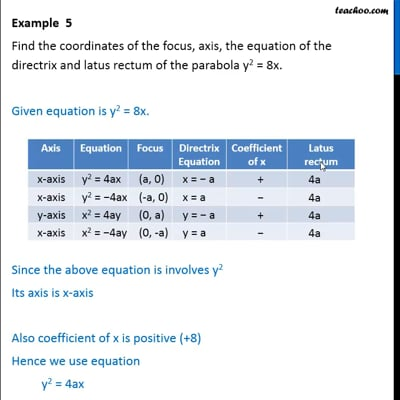Parabola - Basic Questions

Chapter 11 Class 11 Conic Sections
Concept wiseThis video is only available for Teachoo black users

Introducing your new favourite teacher - Teachoo Black, at only ₹83 per month

### Transcript

Example 5 Find the coordinates of the focus, axis, the equation of the directrix and latus rectum of the parabola y2 = 8x. Given equation is y2 = 8x. Since the above equation is involves y2 Its axis is x-axis Also coefficient of x is positive (+8) Hence we use equation y2 = 4ax Comparing y2 = 8x & y2 = 4ax From (1) & (2) 8x = 4ax ﷐8𝑥﷮4𝑥﷯ = a 2 = a a = 2 Coordinates of focus is = (a, 0) = (2, 0) Equation of Directix is x = −a x = −2 Latus Rectum is 4a = 4 × 2 = 8